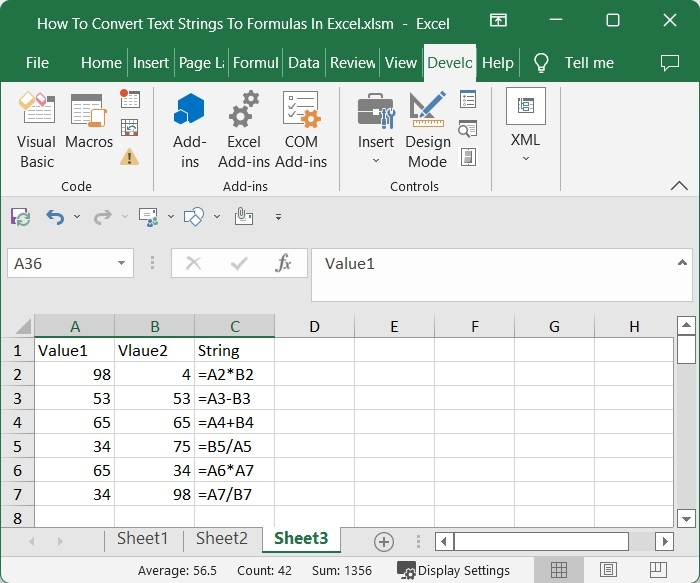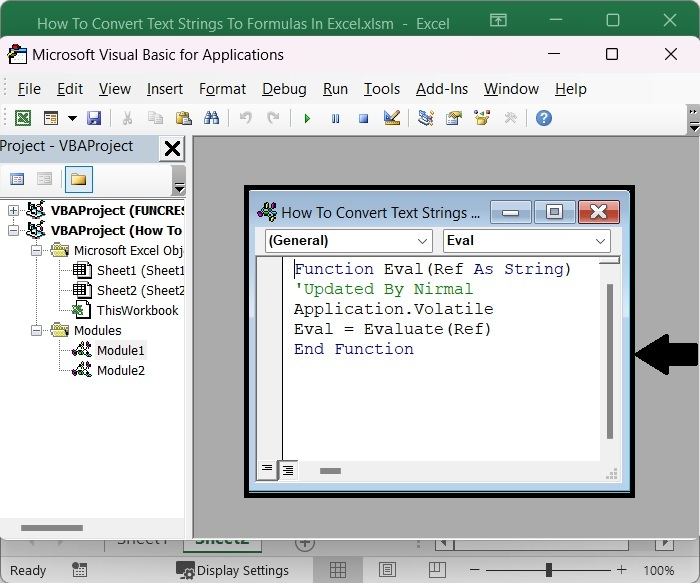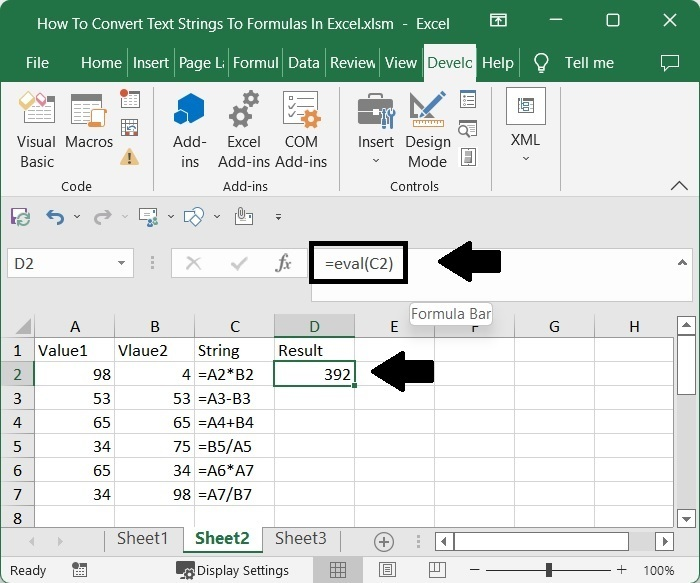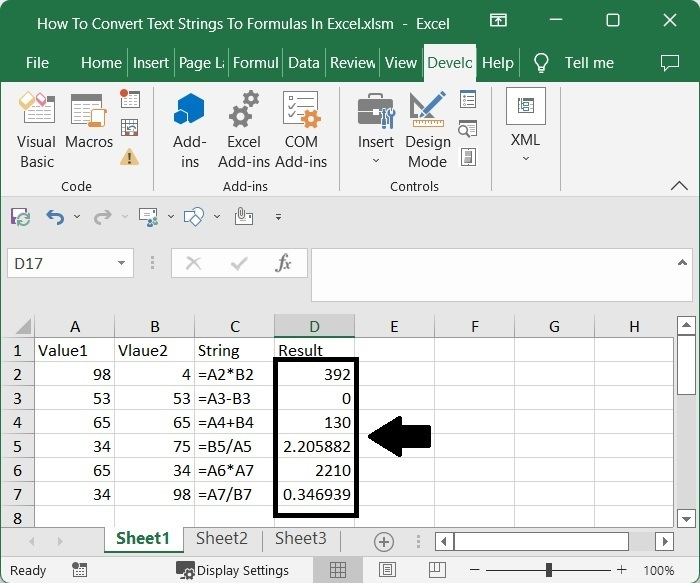# How to Convert Text Strings to Formulas in Excel?

Assume you've saved your formulas as strings in an Excel sheet to be calculated later; if we want to use them, we can because they're stored as strings rather than formulas. It can be very time consuming to manually convert them into formulas. So, we can use a user-defined function to complete our task in a faster way.

Read this tutorial to learn how you can convert text strings to formulas in Excel. We can create the user-defined function using the VBA application. Because this task cannot be completed directly in Excel, we must use a combination of VBA application and formulas.

## Converting Text Strings to Formulas in Excel

In this case, first we will open the VB application, then insert a module with code, run it, and use the created eval formula to complete the procedure.

### Step 1

Consider an excel sheet in which the data is in the form of a table with formulas as strings, as shown in the image below.Now right-click on the sheet name and select View Code to open the VBA application.

Right click > View code

### Step 2

In the VBA application, click on "Insert" and select "Module," then enter the following program code in the text box as shown in the below image.

Insert > Module > Program

### Program

Function Eval(Ref As String)
'Updated By Nirmal
Application.Volatile
Eval = Evaluate(Ref)
End Function### Step 3

Then, using the Alt + Q command, save the sheet as a macro-enabled workbook and exit the vba application. Then, in our case, cell D2, click on an empty cell, enter the formula =eval(C2), and press enter to get our first result, as shown in the image below. In the formula, C2 is the address of the string on the sheet.

Save > Alt + Q > Empty cell > Enter### Step 4

To get all the results, drag down from the first result using the auto-fill handle, and our final result will be similar to the below image.## Conclusion

In this tutorial, we used a simple example to demonstrate how you can convert a text string to a formula in Excel.

Updated on: 06-Mar-2023

9K+ Views